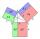# Side c

In △ABC a=2, b=4 and ∠C=100°.

Calculate length of the side c.

Result

c =  4.77

#### Solution:Try calculation via our triangle calculator.

Leave us a comment of example and its solution (i.e. if it is still somewhat unclear...):

Showing 0 comments:Be the first to comment!#### To solve this verbal math problem are needed these knowledge from mathematics:

Do you want to convert length units? Cosine rule uses trigonometric SAS triangle calculator. See also our trigonometric triangle calculator.

## Next similar examples:

1. DiagonalsCalculate the length of the diagonals of the rhombus if its side is long 5 and one of its internal angle is 80°.
2. Greatest angleCalculate the greatest triangle angle with sides 197, 208, 299.
3. Four sides of trapezoidIn the trapezoid ABCD is |AB| = 73.6 mm; |BC| = 57 mm; |CD| = 60 mm; |AD| = 58.6 mm. Calculate the size of its interior angles.
4. In a 2In a thirteen sided polygon, the sum of five angles is 1274°, four of the eight angles remaining are equal and the other four are 18° less than each of the equal angles. Find the angles. .
5. Diagonals in diamondIn the rhombus is given a = 160 cm, alpha = 60 degrees. Calculate the length of the diagonals.
6. Angles by cosine lawCalculate the size of the angles of the triangle ABC, if it is given by: a = 3 cm; b = 5 cm; c = 7 cm (use the sine and cosine theorem).
7. Scalar dot productCalculate u.v if |u| = 5, |v| = 2 and when angle between the vectors u, v is: a) 60° b) 45° c) 120°
8. Clock faceclock face is given. Numbers 10 and 5, and 3 and 8 are connected by straight lines. Calculate the size of their angles.
9. Scalene triangleSolve the triangle: A = 50°, b = 13, c = 6
10. ABCDAC= 40cm , angle DAB=38 , angle DCB=58 , angle DBC=90 , DB is perpendicular on AC , find BD and AD
11. Trapezium ABCDIn the figure, ABDC is a trapezium in which AB || CD. line segments RN and LM are drawn parallel to AB such that AJ=JK=KP. If AB=0.5m and AP=BQ=1.8m, find the lengths of AC, BD, RN and LM. angle D=angle C=60
12. The pondWe can see the pond at an angle 65°37'. Its end points are 155 m and 177 m away from the observer. What is the width of the pond?
13. AnglesIn the triangle ABC, the ratio of angles is: a:b = 4: 5. The angle c is 36°. How big are the angles a, b?
14. Reference angleFind the reference angle of each angle:
15. Vector sumThe magnitude of the vector u is 12 and the magnitude of the vector v is 8. Angle between vectors is 61°. What is the magnitude of the vector u + v?
16. LawsFrom which law follows directly the validity of Pythagoras' theorem in the right triangle? ?
17. Theorem proveWe want to prove the sentence: If the natural number n is divisible by six, then n is divisible by three. From what assumption we started?